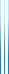# PublicationsPapers

## Asymptotic redundancy and prolixity

Yuval Dagan, Yuval Filmus and Shay Moran
Manuscript

Given a distribution $\mu$ and a set of queries $Q$ (for example, all comparison queries), the cost of $Q$ on $\mu$ is the average depth of a decision tree that locates an item in the support of $\mu$ using only queries from $Q$.

The cost of any set of queries on $\mu$ is always at least the entropy $H(\mu)$, and the redundancy of $Q$ is the maximal gap between the cost of $Q$ on a distribution and its entropy.

The prolixity of a set of queries is defined in the same way, with the entropy replaced by the cost of the optimal unrestricted decision tree.

The redundancy and prolixity of a set of queries is often achieved on degenerate distributions which have very low entropy, and this suggests studying their asymptotic variants, in which we consider distributions whose min-entropy tends to infinity.

Gallager showed that the asymptotic redundancy of unrestricted decision trees is 0.086.

We obtain bounds, and in some case determine, the non-asymptotic and asymptotic redundancy and prolixity of several sets of queries, including comparison queries, comparison and equality queries, and interval queries.

The results in this paper are extracted from the full version of our STOC paper.